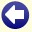## Solution to: The Truel

The solution is C.

The following is given:

• P(A hits) = 1
• P(A misses) = 0
• P(B hits) = 4/5
• P(B misses) = 1/5
• P(C hits) = 1/2
• P(C misses) = 1/2

We shall use the notation P(S,XYZ) for the probability that S survives when the current shooting order is XYZ.

First, calculate the survival chances when only two persons are left.

 Shooting order: Survival chance A: Survival chance B: Survival chance C: Explanation: AB P(A,AB) = 1 P(B,AB) = 0 P(C,AB) = 0 A never misses. AC P(A,AC) = 1 P(B,AC) = 0 P(C,AC) = 0 A never misses. BA P(A,BA) = 1/5 P(B,BA) = 4/5 P(C,BA) = 0 P(A,BA) = P(B misses) × P(A,AB) = 1/5. P(B,BA) = P(B hits) + P(B misses) × P(B,AB) = 4/5. BC P(A,BC) = 0 P(B,BC) = 8/9 P(C,BC) = 1/9 P(B,BC) = P(B hits) + P(B misses) × P(C misses) × P(B,BC) = 4/5 + 1/5 × 1/2 × P(B,BC), which gives P(B,BC) = 8/9. P(C,BC) = P(B misses) × P(C hits) + P(B misses) × P(C misses) × P(C,BC) = 1/5 × 1/2 + 1/5 × 1/2 × P(C,BC), which gives P(C,BC) = 1/9. CA P(A,CA) = 1/2 P(B,CA) = 0 P(C,CA) = 1/2 P(A,CA) = P(C misses) = 1/2. P(C,CA) = P(C hits) = 1/2. CB P(A,CB) = 0 P(B,CB) = 4/9 P(C,CB) = 5/9 P(B,CB) = P(C misses) × P(B hits) + P(C misses) × P(B misses) × P(B,CB) = 1/2 × 4/5 + 1/2 × 1/5 × P(B,CB), which gives P(B,CB) = 4/9. P(C,CB) = P(C hits) + P(C misses) × P(B misses) × P(C,CB) = 1/2 + 1/2 × 1/5 × P(C,CB), which gives P(C,CB) = 5/9.

Then, calculate the survival chances for three persons. This is done by looking at the survival chances for the first shooter, for all four strategies from which he can choose. In bold, the best strategies are indicated.

 Shooting order: Survival chance when shooting at A: Survival chance when shooting at B: Survival chance when shooting at C: Survival chance when missing deliberately: Conclusion: ABC 0 P(A hits) × P(A,CA) + P(A misses) × P(A,BCA) = 1/2 P(A hits) × P(A,BA) + P(A misses) × P(A,BCA) = 1/5 P(A,BCA) < 1/2 (since B will definitely shoot at A, because P(B,AB) = 0!) So, A shoots B, which means that P(A,ABC) = 1/2, P(B,ABC) = 0, and P(C,ABC) = P(C,CA) = 1/2 ACB 0 P(A hits) × P(A,CA) + P(A misses) × P(A,BCA) = 1/2 P(A hits) × P(A,BA) + P(A misses) × P(A,BCA) = 1/5 P(A,CBA) < 1/2 (since B will definitely shoot at A, because P(B,ABC) = 0!) So, A shoots B, which means that P(A,ACB) = 1/2, P(B,ACB) = 0, and P(C,ACB) = P(C,CA) = 1/2 BAC P(B hits) × P(B,CB) + P(B misses) × P(B,ACB) = 16/45 0 P(B hits) × P(B,AB) + P(B misses) × P(B,ACB) = 0 P(B,ACB) = 0 Therefore, B shoots A, which means that P(B,BAC) = 16/45, P(A,BAC) = P(B misses) × P(A,ACB) = 1/10, and P(C,BAC) = P(B hits) × P(C,CB) + P(B misses) × P(C,ACB) = 49/90 CAB P(C hits) × P(C,BC) + P(C misses) × P(C,ABC) = 11/36 P(C hits) × P(C,AC) + P(C misses) × P(C,ABC) = 1/4 0 P(C,ABC) = 1/2 So, C should miss deliberately (fire "into the air"), which means that P(C,CAB) = 1/2, P(A,CAB) = P(A,ABC) = 1/2, and P(B,CAB) = P(B,ABC) = 0 BCA P(B hits) × P(B,CB) + P(B misses) × P(B,CAB) = 16/45 0 P(B hits) × P(B,AB) + P(B misses) × P(B,CAB) = 0 P(B,CAB) = 0 Therefore, B shoots A, which means that P(B,BCA) = 16/45, P(A,BCA) = P(B misses) × P(A,CAB) = 1/10, and P(C,BCA) = P(B hits) × P(C,CB) + P(B misses) × P(C,CAB) = 49/90 CBA P(C hits) × P(C,BC) + P(C misses) × P(C,BAC) = 59/180 P(C hits) × P(C,AC) + P(C misses) × P(C,BAC) = 49/180 0 P(C,BAC) = 49/90 So, C should miss deliberately (fire "into the air"), which means that P(C,CBA) = 49/90, P(A,CBA) = P(A,BAC) = 1/10, and P(B,CBA) = P(B,BAC) = 16/45

This gives the following survival chances for A, B, and C:

 Shooting order: Survival chance of A: Survival chance of B: Survival chance of C: ABC P(A,ABC) = 1/2 P(B,ABC) = 0 P(C,ABC) = 1/2 ACB P(A,ACB) = 1/2 P(B,ACB) = 0 P(C,ACB) = 1/2 BAC P(A,BAC) = 1/10 P(B,BAC) = 16/45 P(C,BAC) = 49/90 CAB P(A,CAB) = 1/2 P(B,CAB) = 0 P(C,CAB) = 1/2 BCA P(A,BCA) = 1/10 P(B,BCA) = 16/45 P(C,BCA) = 49/90 CBA P(A,CBA) = 1/10 P(B,CBA) = 16/45 P(C,CBA) = 49/90 Total survival chances (sum of the probabilities divided by 6): 27/90 16/90 47/90

Conclusion: C has the best chance (47/90) of surviving the duel.Back to the puzzle
This website uses cookies. By further use of this website, or by clicking on 'Continue', you give permission for the use of cookies. If you want more information, look at our cookie policy.